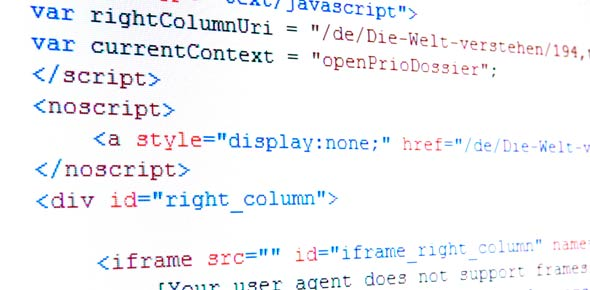# Object Oriented Programming Quiz

20 Questions | Total Attempts: 91SettingsCreate your own Quiz.

• 1.
Which of the following concepts provides facility of using object of one class inside another class?
• A.

Encapsulation

• B.

Inheritance

• C.

Composition

• D.

Abstraction

• 2.
cout is a/an __________ .
• A.

Operator

• B.

Function

• C.

Macro

• D.

Object

• 3.
Which of the following is not a type of constructor?
• A.

Copy constructor

• B.

Default constructor

• C.

Friend constructor

• D.

Parameterized constructor

• 4.
Which of the following type of class allows only one object of it to be created?
• A.

Abstract

• B.

Virtual

• C.

Singleton

• D.

Friend

• 5.
Which of the following concepts means determining at runtime what method to invoke?
• A.

Data hiding

• B.

Dynamic binding

• C.

• D.

Dynamic typing

• 6.
How "Late binding" is implemented in C++?
• A.

Using c++ tables

• B.

Using virtual tables

• C.

Using indexed virtual table

• D.

using polymorphic tables

• 7.
Which of the following operator is overloaded for object cout?
• A.

<<

• B.

>>

• C.

+

• D.

=

• 8.
Which of the following is the correct way of declaring a function as constant?
• A.

Const int ShowData(void) { /* statements */ }

• B.

Int const ShowData(void) { /* statements */ }

• C.

Int ShowData(void) const { /* statements */ }

• D.

Both A and B

• 9.
Which of the following ways are legal to access a class data member using this pointer?
• A.

This->x

• B.

This.x

• C.

*this.x

• D.

*this-x

• 10.
Which of the following is correct about the statements given below?
1. All operators can be overloaded in C++.
2. We can change the basic meaning of an operator in C++.
• A.

Only 1 is true

• B.

Only 2 is true

• C.

Both 1 and 2 are true

• D.

Both are false

• 11.
Which of the following are available only in the class hierarchy chain?
• A.

Public  data members

• B.

Private data member

• C.

Protected Data members

• D.

Member Function

• 12.
Which of the following is not a type of inheritance?
• A.

Multiple

• B.

Multilevel

• C.

Distributive

• D.

Hierarchical

• 13.
What happens if the base and derived class contains definition of a function with same prototype?
• A.

Compiler reports an error on compilation.

• B.

Only base class function will get called irrespective of object.

• C.

Only derived class function will get called irrespective of object.

• D.

Base class object will call base class function and derived class object will call derived class function.

• 14.
Which of the following statements is correct in C++?
• A.

Classes cannot have data as protected members.

• B.

Structures can have functions as members.

• C.

Class members are public by default.

• D.

Structure members are private by default.

• 15.
Which of the following access specifier is used as a default in a class definition?
• A.

Protected

• B.

Public

• C.

Private

• 16.
What is correct about the static data member of a class?
• A.

A static member function can access only static data members of a class.

• B.

A static data member is shared among all the object of the class.

• C.

A static data member can be accessed directly from main().

• D.

A static member function can access only static data members of a class and A static data member is shared among all the object of the class.

• 17.
Which of the following provides a reuse mechanism?
• A.

Abstarction

• B.

Inheritence

• C.

Dynamic Binding

• D.

Encapsulation

• 18.
Which of the following statement is correct?
• A.

Class is an instance of object.

• B.

Object is an instance of a class.

• C.

Class is an instance of data type.

• D.

Object is an instance of data type.

• 19.
How many types of polymorphisms are supported by C++?
• A.

1

• B.

2

• C.

3

• D.

4

• 20.
Which of the following is an abstract data type?
• A.

Int

• B.

Class

• C.

String

• D.

Double

Related TopicsBack to top
×

Wait!
Here's an interesting quiz for you.